# T test

10. Jun 2013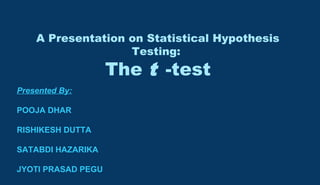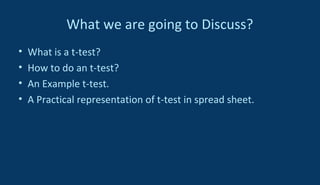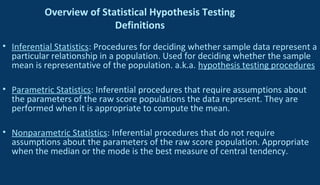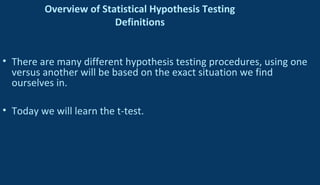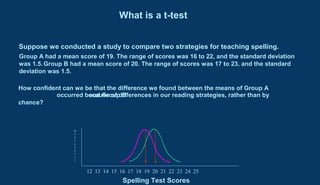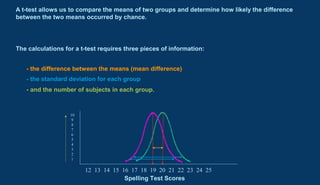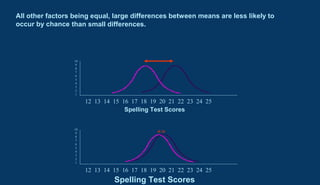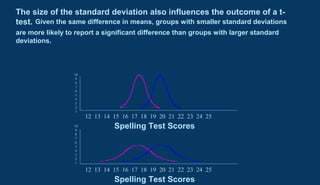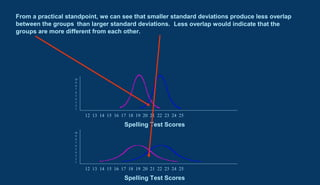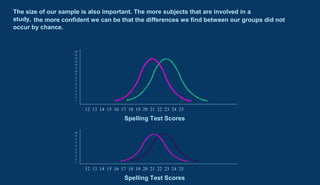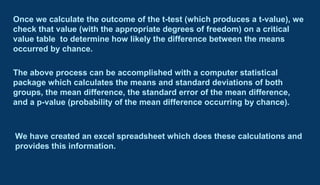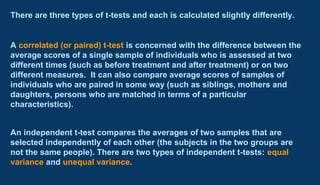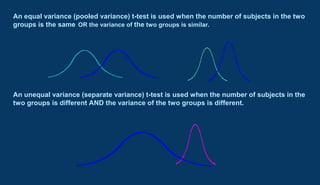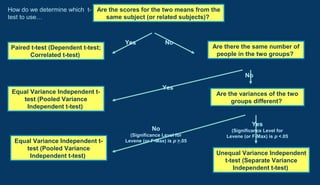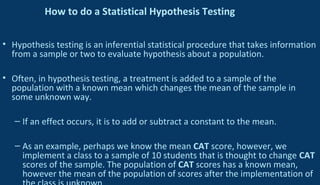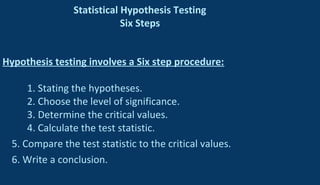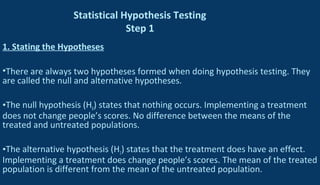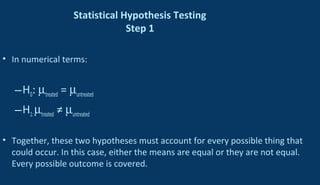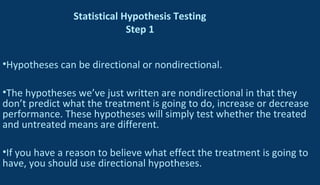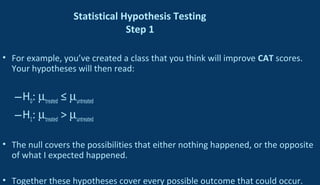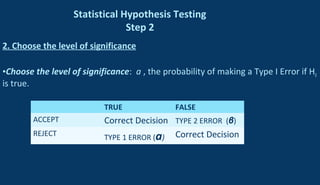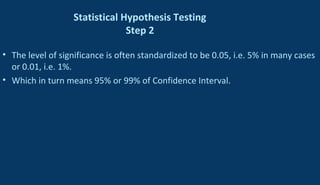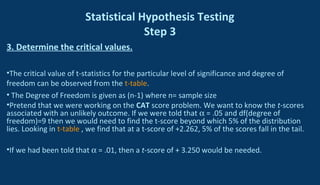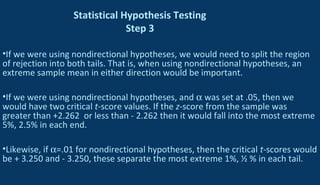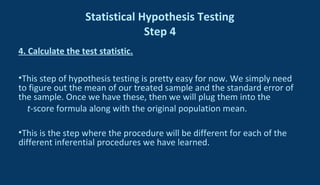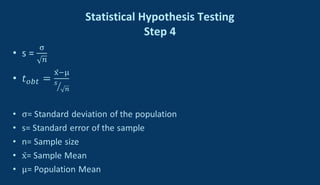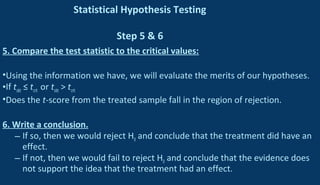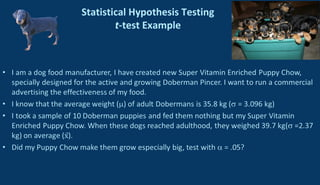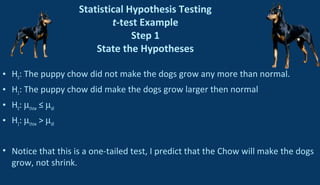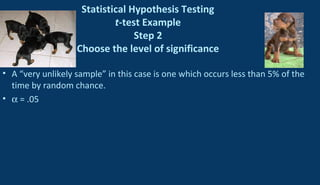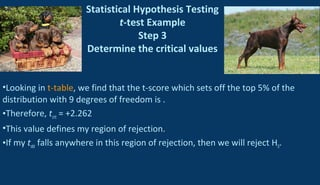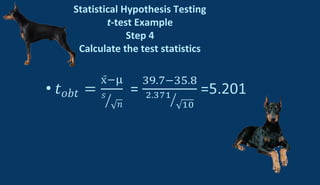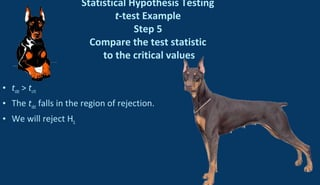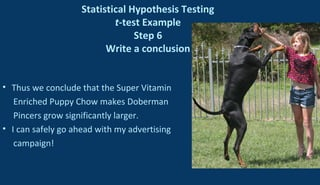1 von 34

### T test

• 1. A Presentation on Statistical Hypothesis Testing: The t -test Presented By: POOJA DHAR RISHIKESH DUTTA SATABDI HAZARIKA JYOTI PRASAD PEGU
• 2. What we are going to Discuss? • What is a t-test? • How to do an t-test? • An Example t-test. • A Practical representation of t-test in spread sheet.
• 3. Overview of Statistical Hypothesis Testing Definitions • Inferential Statistics: Procedures for deciding whether sample data represent a particular relationship in a population. Used for deciding whether the sample mean is representative of the population. a.k.a. hypothesis testing procedures • Parametric Statistics: Inferential procedures that require assumptions about the parameters of the raw score populations the data represent. They are performed when it is appropriate to compute the mean. • Nonparametric Statistics: Inferential procedures that do not require assumptions about the parameters of the raw score population. Appropriate when the median or the mode is the best measure of central tendency.
• 4. Overview of Statistical Hypothesis Testing Definitions • There are many different hypothesis testing procedures, using one versus another will be based on the exact situation we find ourselves in. • Today we will learn the t-test.
• 5. occurred because of differences in our reading strategies, rather than by chance? 12 13 14 15 16 17 18 19 20 21 22 23 24 25 10 9 8 7 6 5 4 3 2 1 Spelling Test Scores Suppose we conducted a study to compare two strategies for teaching spelling. Group A had a mean score of 19. The range of scores was 16 to 22, and the standard deviation was 1.5.Group B had a mean score of 20. The range of scores was 17 to 23, and the standard deviation was 1.5. How confident can we be that the difference we found between the means of Group A and Group B What is a t-test
• 6. 12 13 14 15 16 17 18 19 20 21 22 23 24 25 10 9 8 7 6 5 4 3 2 1 Spelling Test Scores A t-test allows us to compare the means of two groups and determine how likely the difference between the two means occurred by chance. The calculations for a t-test requires three pieces of information: - the difference between the means (mean difference) - the standard deviation for each group - and the number of subjects in each group.
• 7. All other factors being equal, large differences between means are less likely to occur by chance than small differences. 12 13 14 15 16 17 18 19 20 21 22 23 24 25 10 9 8 7 6 5 4 3 2 1 Spelling Test Scores 12 13 14 15 16 17 18 19 20 21 22 23 24 25 10 9 8 7 6 5 4 3 2 1 Spelling Test Scores
• 8. 12 13 14 15 16 17 18 19 20 21 22 23 24 25 10 9 8 7 6 5 4 3 2 1 Spelling Test Scores 12 13 14 15 16 17 18 19 20 21 22 23 24 25 10 9 8 7 6 5 4 3 2 1 Spelling Test Scores The size of the standard deviation also influences the outcome of a t- test. Given the same difference in means, groups with smaller standard deviations are more likely to report a significant difference than groups with larger standard deviations.
• 9. Less overlap would indicate that the groups are more different from each other. than larger standard deviations. From a practical standpoint, we can see that smaller standard deviations produce less overlap between the groups 12 13 14 15 16 17 18 19 20 21 22 23 24 25 10 9 8 7 6 5 4 3 2 1 Spelling Test Scores 12 13 14 15 16 17 18 19 20 21 22 23 24 25 10 9 8 7 6 5 4 3 2 1 Spelling Test Scores
• 10. 12 13 14 15 16 17 18 19 20 21 22 23 24 25 10 9 8 7 6 5 4 3 2 1 Spelling Test Scores The size of our sample is also important. The more subjects that are involved in a study, 12 13 14 15 16 17 18 19 20 21 22 23 24 25 16 15 14 13 12 11 10 9 8 7 6 5 4 3 2 1 Spelling Test Scores the more confident we can be that the differences we find between our groups did not occur by chance.
• 11. Once we calculate the outcome of the t-test (which produces a t-value), we check that value (with the appropriate degrees of freedom) on a critical value table to determine how likely the difference between the means occurred by chance. The above process can be accomplished with a computer statistical package which calculates the means and standard deviations of both groups, the mean difference, the standard error of the mean difference, and a p-value (probability of the mean difference occurring by chance). We have created an excel spreadsheet which does these calculations and provides this information.
• 12. A correlated (or paired) t-test is concerned with the difference between the average scores of a single sample of individuals who is assessed at two different times (such as before treatment and after treatment) or on two different measures. It can also compare average scores of samples of individuals who are paired in some way (such as siblings, mothers and daughters, persons who are matched in terms of a particular characteristics). An independent t-test compares the averages of two samples that are selected independently of each other (the subjects in the two groups are not the same people). There are two types of independent t-tests: equal variance and unequal variance. There are three types of t-tests and each is calculated slightly differently.
• 13. An equal variance (pooled variance) t-test is used when the number of subjects in the two groups is the same OR the variance of the two groups is similar. An unequal variance (separate variance) t-test is used when the number of subjects in the two groups is different AND the variance of the two groups is different.
• 14. Are the scores for the two means from the same subject (or related subjects)? Paired t-test (Dependent t-test; Correlated t-test) Yes Equal Variance Independent t- test (Pooled Variance Independent t-test) Yes Unequal Variance Independent t-test (Separate Variance Independent t-test) Yes (Significance Level for Levene (or F-Max) is p <.05 Equal Variance Independent t- test (Pooled Variance Independent t-test) No (Significance Level for Levene (or F-Max) is p >.05 Are there the same number of people in the two groups? No Are the variances of the two groups different? No How do we determine which t- test to use…
• 15. How to do a Statistical Hypothesis Testing • Hypothesis testing is an inferential statistical procedure that takes information from a sample or two to evaluate hypothesis about a population. • Often, in hypothesis testing, a treatment is added to a sample of the population with a known mean which changes the mean of the sample in some unknown way. – If an effect occurs, it is to add or subtract a constant to the mean. – As an example, perhaps we know the mean CAT score, however, we implement a class to a sample of 10 students that is thought to change CAT scores of the sample. The population of CAT scores has a known mean, however the mean of the population of scores after the implementation of
• 16. Statistical Hypothesis Testing Six Steps Hypothesis testing involves a Six step procedure: 1. Stating the hypotheses. 2. Choose the level of significance. 3. Determine the critical values. 4. Calculate the test statistic. 5. Compare the test statistic to the critical values. 6. Write a conclusion.
• 17. Statistical Hypothesis Testing Step 1 1. Stating the Hypotheses •There are always two hypotheses formed when doing hypothesis testing. They are called the null and alternative hypotheses. •The null hypothesis (H0) states that nothing occurs. Implementing a treatment does not change people’s scores. No difference between the means of the treated and untreated populations. •The alternative hypothesis (H1) states that the treatment does have an effect. Implementing a treatment does change people’s scores. The mean of the treated population is different from the mean of the untreated population.
• 18. Statistical Hypothesis Testing Step 1 • In numerical terms: –H0: µtreated = µuntreated –H1:µtreated ≠ µuntreated • Together, these two hypotheses must account for every possible thing that could occur. In this case, either the means are equal or they are not equal. Every possible outcome is covered.
• 19. Statistical Hypothesis Testing Step 1 •Hypotheses can be directional or nondirectional. •The hypotheses we’ve just written are nondirectional in that they don’t predict what the treatment is going to do, increase or decrease performance. These hypotheses will simply test whether the treated and untreated means are different. •If you have a reason to believe what effect the treatment is going to have, you should use directional hypotheses.
• 20. Statistical Hypothesis Testing Step 1 • For example, you’ve created a class that you think will improve CAT scores. Your hypotheses will then read: –H0: µtreated ≤ µuntreated –H1: µtreated > µuntreated • The null covers the possibilities that either nothing happened, or the opposite of what I expected happened. • Together these hypotheses cover every possible outcome that could occur.
• 21. Statistical Hypothesis Testing Step 2 2. Choose the level of significance •Choose the level of significance: a , the probability of making a Type I Error if H0 is true. TRUE FALSE ACCEPT Correct Decision TYPE 2 ERROR (β) REJECT TYPE 1 ERROR (a) Correct Decision
• 22. Statistical Hypothesis Testing Step 2 • The level of significance is often standardized to be 0.05, i.e. 5% in many cases or 0.01, i.e. 1%. • Which in turn means 95% or 99% of Confidence Interval.
• 23. Statistical Hypothesis Testing Step 3 3. Determine the critical values. •The critical value of t-statistics for the particular level of significance and degree of freedom can be observed from the t-table. • The Degree of Freedom is given as (n-1) where n= sample size •Pretend that we were working on the CAT score problem. We want to know the t-scores associated with an unlikely outcome. If we were told that α = .05 and df(degree of freedom)=9 then we would need to find the t-score beyond which 5% of the distribution lies. Looking in t-table , we find that at a t-score of +2.262, 5% of the scores fall in the tail. •If we had been told that α = .01, then a t-score of + 3.250 would be needed.
• 24. Statistical Hypothesis Testing Step 3 •If we were using nondirectional hypotheses, we would need to split the region of rejection into both tails. That is, when using nondirectional hypotheses, an extreme sample mean in either direction would be important. •If we were using nondirectional hypotheses, and α was set at .05, then we would have two critical t-score values. If the z-score from the sample was greater than +2.262 or less than - 2.262 then it would fall into the most extreme 5%, 2.5% in each end. •Likewise, if α=.01 for nondirectional hypotheses, then the critical t-scores would be + 3.250 and - 3.250, these separate the most extreme 1%, ½ % in each tail.
• 25. Statistical Hypothesis Testing Step 4 4. Calculate the test statistic. •This step of hypothesis testing is pretty easy for now. We simply need to figure out the mean of our treated sample and the standard error of the sample. Once we have these, then we will plug them into the t-score formula along with the original population mean. •This is the step where the procedure will be different for each of the different inferential procedures we have learned.
• 26. Statistical Hypothesis Testing Step 4
• 27. Statistical Hypothesis Testing Step 5 & 6 5. Compare the test statistic to the critical values: •Using the information we have, we will evaluate the merits of our hypotheses. •If tobt ≤ tcrit or tobt > tcrit •Does the t-score from the treated sample fall in the region of rejection. 6. Write a conclusion. – If so, then we would reject H0 and conclude that the treatment did have an effect. – If not, then we would fail to reject H0 and conclude that the evidence does not support the idea that the treatment had an effect.
• 28. Statistical Hypothesis Testing t-test Example
• 29. Statistical Hypothesis Testing t-test Example Step 1 State the Hypotheses • H0: The puppy chow did not make the dogs grow any more than normal. • H1: The puppy chow did make the dogs grow larger then normal • H0: µchow ≤ µall • H1: µchow > µall • Notice that this is a one-tailed test, I predict that the Chow will make the dogs grow, not shrink.
• 30. Statistical Hypothesis Testing t-test Example Step 2 Choose the level of significance • A “very unlikely sample” in this case is one which occurs less than 5% of the time by random chance. • α = .05
• 31. Statistical Hypothesis Testing t-test Example Step 3 Determine the critical values •Looking in t-table, we find that the t-score which sets off the top 5% of the distribution with 9 degrees of freedom is . •Therefore, tcrit = +2.262 •This value defines my region of rejection. •If my tobt falls anywhere in this region of rejection, then we will reject H0.
• 32. Statistical Hypothesis Testing t-test Example Step 4 Calculate the test statistics
• 33. Statistical Hypothesis Testing t-test Example Step 5 Compare the test statistic to the critical values • tobt > tcrit • The tobt falls in the region of rejection. • We will reject H0.
• 34. Statistical Hypothesis Testing t-test Example Step 6 Write a conclusion • Thus we conclude that the Super Vitamin Enriched Puppy Chow makes Doberman Pincers grow significantly larger. • I can safely go ahead with my advertising campaign!

### Hinweis der Redaktion

1. Do homework 6-1, 6-2, &amp; 6-3
2. -Our job with the rest of hypothesis testing is to find which of these hypotheses the evidence supports.
3. -(Draw a distribution of sample means, using SAT scores as an example.) -(Draw a second distribution of sample means to the right of the original one.)
4. -Draw a distribution with 2.262 setting off the top 5%, draw the region of rejection.
5. -Draw a distribution with 2.262 setting off the top 5%, draw the region of rejection.
6. -Draw the location of the obtained z score.
7. -Draw the location of the obtained z score.